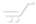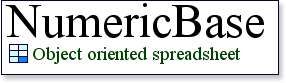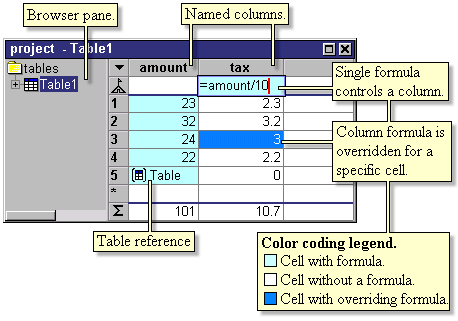# Numericbase

NumericBase is an experimental spreadsheet program with a unique method of creating solutions. This object-oriented method replaces the repetitive-formula approach of other spreadsheets.

• Solve difficult problems. If you have difficulties solving a particular problem using other spreadsheets,then NumericBase unique approach may help you solve your problem faster.
• Understand your solutions. Solutions created using NumericBase are easier to understand. This means that you can understand, and improve, solutions that you created long time ago, or even solutions created by other people.
• Create more flexible solutions. You can modify basic parameters of your solution by modifying a single formula, rather than modifying many repetitive formulas . For example, a multiplication table that you can change to addition table, by modifying just one formula.

Screenshot
Some of NumericBase’s features are illustrated in the following
screenshot, showing a portion of NumericBase display (yellow notes are clickable).Screenshot: Illustration of NumericBase
features.

Basic principles
When building a solution with NumericBase you can(among other):

• Use a single formula to control the values of all the cells across a column.
• Override this single formula for a specific cell.
• Refer to other cells using a meaningful column and row:row_names_and_column_numbers.htm names.
• Understand the solution structure using the color-coding ,
source code, and browser features.

More features

Using NumericBase you can also:

Getting started
To get stated you can: